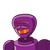# A die is rolled is the probability that the number appearing on upper face is less than 3​

A die is rolled is the probability that the number appearing on upper face is less than 3​

### 1 thought on “A die is rolled is the probability that the number appearing on upper face is less than 3​”

1.Step-by-step explanation:

Probability of number appearing less than 3 =

2/6 or 1/3